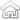The following formula calculates the power used by any electric device.

For example:
An ordinary light bulb, (100Watt) uses in 1 hour:
100Watt * 1 Hour = 100Wh (Watt Hours)

While an "economical" one of 15 Watt uses in 1 hour:
15Watt *1 Hour = 15 Wh (Watt Hours)

 Power         *     Hours of use      =   Energy (the  Watt)    (multiplied)